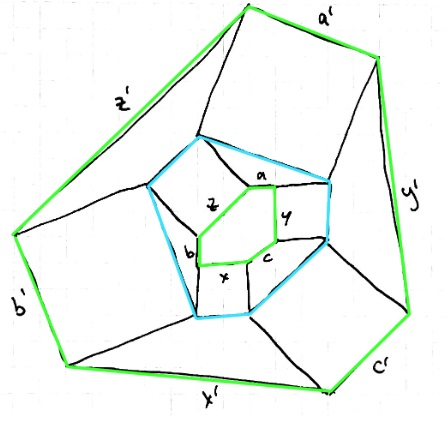# Exploring Vecten's Wreaths with Linear Algebra

### Introduction

What follows is Long Huynh Huu's development of a result by Hirotaka Ebisui and Thanos Kalogerakis, reported elsewhere earlier.

The problem deals with an extension of Vecten's construction of squares on the sides of a triangle and then adding another layer of squares and so on.

Hirotaka Ebisui has shown that, if the first layer of squares starts at a right triangle, then a strange identity pops up:Hirotaka Ebisui has named his result the Pythagorean Fivefold Theorem, of which I was kindly informed by Thanos Kalogerakis who observed in private correspondence that the next layer of squares appears to obey the regular Pythagorean relation.

Long Huynh Huu took it from there.

### Findings

1. It suffices to know the progression of polygons which are the convex hull of every second layer. In the following image you see them in green:Every square shares a side with one polygon.

2. The initial triangle does not need to be a right triangle. In fact we will see that $\textbf{any}$ linear relation between $A,B,C$ will hold for the subsequent triples of squares:

\begin{align} &\lambda_1 A + \lambda_2 B + \lambda_3 C = 0 \\\Rightarrow& \lambda_1 A'' + \lambda_2 B'' + \lambda_3 C'' = 0 \\\Rightarrow& \lambda_1 A'''' + \lambda_2 B'''' + \lambda_3 C'''' = 0 \\\Rightarrow& ... \end{align}

and similarly

\begin{align} &\mu_1 A' + \mu_2 B' + \mu_3 C' = 0 \\\Rightarrow& \mu_1 A''' + \mu_2 B''' + \mu_3 C''' = 0 \\\Rightarrow& ... \end{align}

3. The sizes of the squares grow according to a recursion formula.

### Proof

So let's dive in. The green polygons have six sides which I represent as vectors/complex numbers: $a,b,c,x,y,z \in \mathbb{C}$.For a given green polygon, its $\textbf{successor}$ $(a',b',c',x',y',z')$ is given by the following construction:Without too much headache, we can compute this successor in two steps (just as we constructed the squares in two steps):

$\textbf{L}_1:$ The intermediate (blue) polygon in above figure has sides $(x,y,z)$ and $(a',b',c')$:

\begin{align} a' =& iy + a - iz \\ b' =& iz + b - ix \\ c' =& ix + c - iy \\ \end{align}

Therefore

$\begin{pmatrix} a'\\b'\\c'\\x\\y\\z \end{pmatrix} = \underset{L_1}{ \underbrace{ \left[ \begin{array}{cccccc} 1 & 0 & 0 & 0 & i & -i \\ 0 & 1 & 0 & i & -i & 0 \\ 0 & 0 & 1 & -i & 0 & i \\ 0 & 0 & 0 & 1 & 0 & 0 \\ 0 & 0 & 0 & 0 & 1 & 0 \\ 0 & 0 & 0 & 0 & 0 & 1 \\ \end{array} \right]}} \begin{pmatrix} a\\b\\c\\x\\y\\z \end{pmatrix}$

$\textbf{L}_2:$ We get from the intermediate polygon to the outer polygon via

\begin{align} x' =& ib' + x -ic' \\ y' =& ic' + y -ia' \\ z' =& ia' + z -ib' \\ \end{align}

Therefore

$\begin{pmatrix} a'\\b'\\c'\\x'\\y'\\z' \end{pmatrix} = \underset{L_2}{ \underbrace{ \left[ \begin{array}{cccccc} 1 & 0 & 0 & 0 & 0 & 0 \\ 0 & 1 & 0 & 0 & 0 & 0 \\ 0 & 0 & 1 & 0 & 0 & 0 \\ 0 & -i & i & 1 & 0 & 0 \\ -i & i & 0 & 0 & 1 & 0 \\ i & 0 & -i & 0 & 0 & 1 \\ \end{array} \right]}} \begin{pmatrix} a'\\b'\\c'\\x\\y\\z \end{pmatrix}$

Hence to get the outer polygon, we need to compose $L_2$ and $L_1$ and apply this to $(a,b,c,x,y,z)^t$. Before we continue let's define some matrices which will make the following computations easy:

\begin{align} I :=& \begin{pmatrix} 1&0&0\\0&1&0\\0&0&1 \end{pmatrix} \\ E :=& \begin{pmatrix} 1&1&1\\1&1&1\\1&1&1 \end{pmatrix} \\ T :=& \begin{pmatrix} 0&i&-i\\i&-i&0\\-i&0&i \end{pmatrix} \\ -TT =& 3I-E \end{align}

Now we define our $\textbf{successor matrix}:$

$S := L_2L_1 = \begin{pmatrix} I&0\\-T&I \end{pmatrix} \begin{pmatrix} I&T\\0&I \end{pmatrix} = \begin{pmatrix} I&T\\-T&4I-E \end{pmatrix}$

As we start with a $\textbf{triangle},$ the first polygon has

$a_0,b_0,c_0 = 0 \text{ and } x_0+y_0+z_0 = 0.$

The $n^{th}$ outer polygon is $S^n(0,0,0,x_0,y_0,z_0)^t =: (a_n,b_n,c_n,x_n,y_n,z_n)^t$.

One thing you can observe when computing examples is that $w^n := (x_n,y_n,z_n)^t$ and $w^0$ are collinear, and so are $v^n := (a_n,b_n,c_n)^t$ and $v^1.$

### Claim

There exists sequences $\{\lambda_n\}, \{\mu_n\}$ such that

$\begin{pmatrix} v^n\\w^n \end{pmatrix} = \begin{pmatrix} \lambda_n v^1 \\ \mu_n w^0 \end{pmatrix}$

for $n \geq 0$. The sequence is $\textbf{independent of the initial triangle}$ $(x_0,y_0,z_0)$ and is given by

$\begin{pmatrix} \lambda_0 \\ \mu_0 \end{pmatrix} = \begin{pmatrix} 0 \\ 1 \end{pmatrix} \qquad \begin{pmatrix} \lambda_{n+1} \\ \mu_{n+1} \end{pmatrix} = \begin{pmatrix} \lambda_n + \mu_n \\ 3\lambda_n + 4\mu_n \end{pmatrix}$

### Proof

Clearly

$\begin{pmatrix} \lambda_0 v^1 \\ \mu_0 w^0 \end{pmatrix} = \begin{pmatrix} v^0 \\ w^0 \end{pmatrix}$

Note that $Ew^0 = 0$, as the components of $w^0$ add up to zero, and that $v^1 = Tw^0$. The following induction step then verifies the recursion formula:

\begin{align} S\begin{pmatrix}\lambda_n v^1\\\mu_n w^0 \end{pmatrix} &= \begin{pmatrix} \lambda_n v^1 + \mu_n T w^0 \\ -\lambda_n Tv^1 + 4\mu_n w^0 - \mu_n E w^0 \end{pmatrix}\\ &= \begin{pmatrix} \lambda_n v^1 + \mu_n v^1 \\ -\lambda_n T^2w^0 + 4\mu_n w^0 \end{pmatrix}\\ &= \begin{pmatrix} (\lambda_n + \mu_n) v^1 \\ (3\lambda_n + 4\mu_n) w^0 \end{pmatrix}. \end{align}

Remark: The sequences $\{\lambda_n\}$ and $\{\mu_n\}$ are apparently A004254 and A004253 respectively.

This proves my second finding.

As a corollary, we can conclude the third one too. For example,

$|w^n_1|^2 + |w^n_2|^2 - |w^n_3|^2 = \mu_n^2 (|x_0|^2+|y_0|^2-|z_0|^2),$

so that

$|x_0|^2+|y_0|^2 - |z_0|^2 = 0 \Rightarrow |w^n_1|^2 + |w^n_2|^2 - |w^n_3|^2 = 0$

and

$|v^n_1|^2 + |v^n_2|^2 - 5|v^n_3|^2 = \lambda_n^2 (|a_1|^2+|b_1|^2-5|c_1|^2),$

so that

$|a_1|^2+|b_1|^2 - 5|c_1|^2 = 0 \Rightarrow |v^n_1|^2 + |v^n_2|^2 - 5|v^n_3|^2=0.$